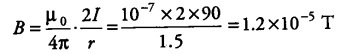# A horizontal overhead power line carries a current of 90 A

A horizontal overhead power line carries a current of 90 A, in East to West direction. What are the magnitude and direction of the magnetic field due to the current 1.5 m below the line?

Given, / = 90 A and r = 1.5 m
Here, point P is below the power line, where we have to find the magnetic field and its direction.The magnitude of magnetic field,The direction of magnetic field is given by right hand thumb rule. So, the direction of magnetic field at point P due to the flowing current is perpendicularly outwards to the plane of paper.